### Home > CALC > Chapter 1 > Lesson 1.2.3 > Problem1-58

1-58.
1. Estimate A(f(x), −3 ≤ x ≤ 3) for f(x) = 2x2 + 1. 1-58 HW eTool (Desmos). Homework Help ✎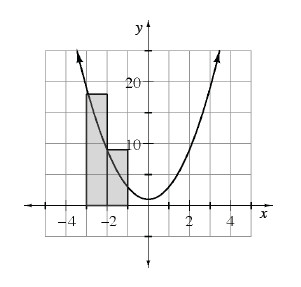1. Using left endpoint rectangles. The first two rectangles are shown.

2. Using right endpoint rectangles.

3. Using trapezoids. What do you notice? Does this always happen?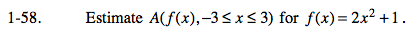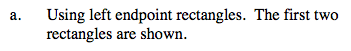Regarding the word ESTIMATE: You are not being asked to make a guess. You still need to compute. Of course, the area using rectangles is an approximation of the actual area under f(x).

Sketch the remaining rectangle. Notice: The height of the LAST rectangle is evaluated at x = 2 (not x = 3).

Each rectangle has a base of 1 and a height of f(x). Area = 1(f(−3) + f(−2) + f(−1) + f(0) + f(1) + f(2)) =_____________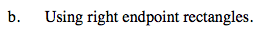Notice: The height of the FIRST rectangle is evaluated at x = −2. The height of the LAST rectangle is evaluated at x = 3.

Each rectangle has a base of 1 and a height of f(x). Area = 1( f(-2) + f(-1) + f(0) + f(1) + f(2) + f(3)) =_____________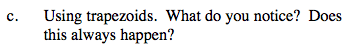The trapezoidal sum is the AVERAGE of the left- and right-endpoint sums! Expain why this happens (algebraically and geometrically).

Use the eTool below to view the graphs.
Click the link at right for the full version of the eTool: Calc 1-58 HW eTool